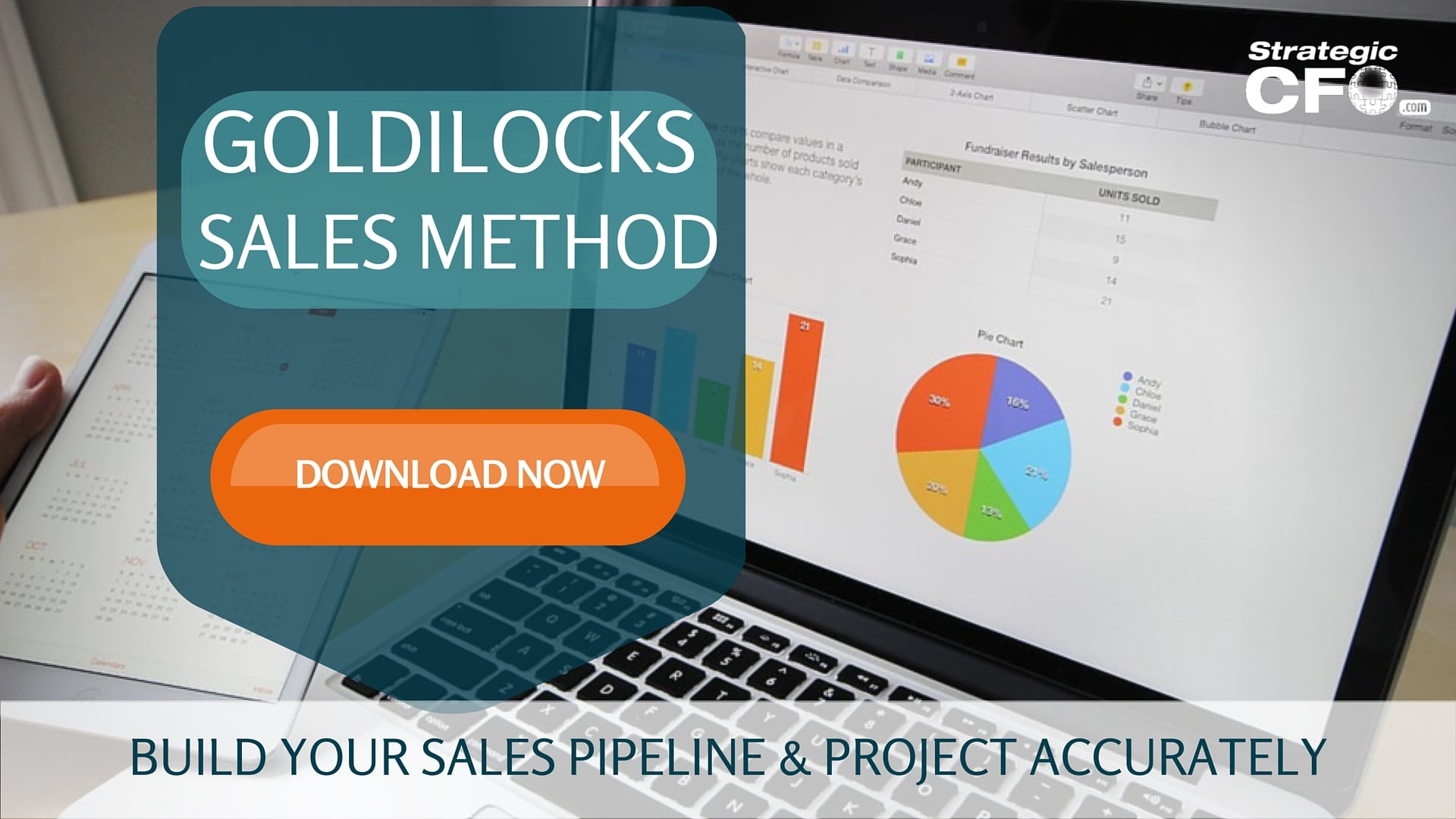# Forecasting in Accounting

Forecasting in accounting refers to the process of using current and historic cost data to predict future costs. Forecasting is important for planning purposes – it is necessary to estimate and plan for costs that will be incurred prior to actually incurring them. There are several common tools and techniques used for forecasting in the field of cost accounting, including the following:

## Budgeting

Budgeting is the process of preparing a budget in order to plan for revenues and expenses in an upcoming fiscal period.

A budget has five primary objectives. These include the following:

1. Planning for the upcoming fiscal period
2. Facilitating coordination and communication of these plans throughout the organization
3. Allocating resources within an organization
4. Managing financial and operational performance during the fiscal period
5. Evaluating performance and providing goal-based incentives to management and other personnel inside the organization

Budgets are prepared using current and historic data and estimations about future trends. Budgets can also be prepared using the traditional method or the zero-based method. The tradition method of budgeting typically uses the previous period’s budget at a starting point for the upcoming period’s budget. The zero-based budget method essentially requires starting from scratch each period.

### High-Low Method

You can use the high-low method is a technique for cost estimation in forecasting. It is a rather simple technique and it is less accurate than more sophisticated cost estimation techniques, such as regression analysis.

Using the high-low method requires having a set of data relating costs to cost-driver activities. You take the highest cost and the highest cost-driver activity level and the lowest cost and the lowest cost-driver activity level from the data set. Then use these four pieces of data to calculate the slope of the line that connects the two points. Finally, you compute the intercept using the slope and one of the points. This gives you the high-low cost equation for that particular cost-incurring activity.

### Regression Analysis

Regression analysis is a method of relating a dependent variable to an independent variable. The analysis essentially computes how much of the variance in the dependent variable is due to variations in the independent variable. Regression analysis requires having a set of data for both the dependent and independent variables. The best way to do a regression analysis is in a computer program.

Regression analysis can be either simple or multiple. Simple regression analysis uses one independent variable and one dependent variable. Whereas, multiple regression analysis uses several independent variables and one dependent variable. The result of a regression analysis is an equation that can be used to forecast costs based on certain estimates of independent variable activity.

If you need help creating an accurate forecast, then download our free Goldilocks Sales Method whitepaper to project accurately.Strategic CFO Lab Member Extra